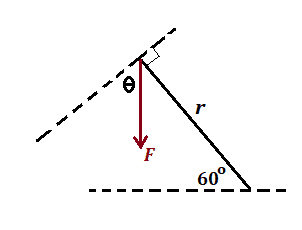# Torques on a vertical wheel due to 3 masses spaced along the wheel rim

paulimerci
Homework Statement:
A wheel of radius R and negligible mass is mounted on a horizontal frictionless axle so that the wheel is in a vertical plane. Three small objects having masses m, M, and 2M, respectively, are mounted on the rim of the wheel, as shown. If the system is in static equilibrium, what is the value of m in terms of M ?
Relevant Equations:
Rotational equilibrium:
T = F × r × sinθ. T = torque. F = linear force. r = distance measured from the axis of rotation to where the application of linear force takes place.
Applying rotational equilibrium at the center pivot we get:
+mg(R) + Mg(Rcos60°)–2Mg(R) = 0.Using cos60° = ½ we arrive at the answer 3M/2

I don't understand why cosine is used instead of sine in the above equation. I see the y component mg is acting perpendicular to the x component and so from the equation of Torque we understand that the force perpendicular to "r" is taken into consideration. I would really appreciate if anyone could explain.

#### Attachments

•Screen Shot 2022-10-28 at 2.15.46 PM.png
19.5 KB · Views: 19

Staff Emeritus
Homework Helper
Gold Member

•topsquark
paulimerci
Thanks, I forgot to insert the picture. I've just uploaded it for your reference .

Homework Helper
Gold Member
2022 Award
There are two ways to think of it:
1. R x the component of Mg perpendicular to R, ##RMg\cos(60)##
2. Mg x the component of R perpendicular to Mg, ##MgR\cos(60)##
Where do you disagree?

•topsquark and paulimerci
paulimerci
Thanks, I find it hard visualizing it.
Why sin 60 is not used in the equation?

Homework Helper
Gold Member
Why sin 60 is not used in the equation?
What is the x-component of the radius from the center to mass ##M##? Is it ##R\cos(60^o)## or ##R\sin(60^o)##?

•topsquark
paulimerci
Well its R cos 60.

paulimerci
What is the x-component of the radius from the center to mass ##M##? Is it ##R\cos(60^o)## or ##R\sin(60^o)##?
While calculating torque for m, which is to the left we take it as sin 90 and thereby for calculating M which is at the top of the circle why cannot we take that as sin 60?

Homework Helper
Gold Member
from the equation of Torque we understand that the force perpendicular to "r" is taken into consideration.What is the value of the angle ##\theta## shown in the figure above? How would you use that to find the component of the force perpendicular to ##r##?

•paulimerci and topsquark
paulimerci
Theta is 60. The angle between r and F is 30. I will resolve F into x and y components. Y component is parallel to r and x component looks perpendicular to r. Was it right?

Homework Helper
Gold Member
•paulimerci
paulimerci
Thank you all
View attachment 316304

What is the value of the angle ##\theta## shown in the figure above? How would you use that to find the component of the force perpendicular to ##r##?
Thank you TSny! This diagram helped me to figure it out.

•Lnewqban and TSny
paulimerci
•